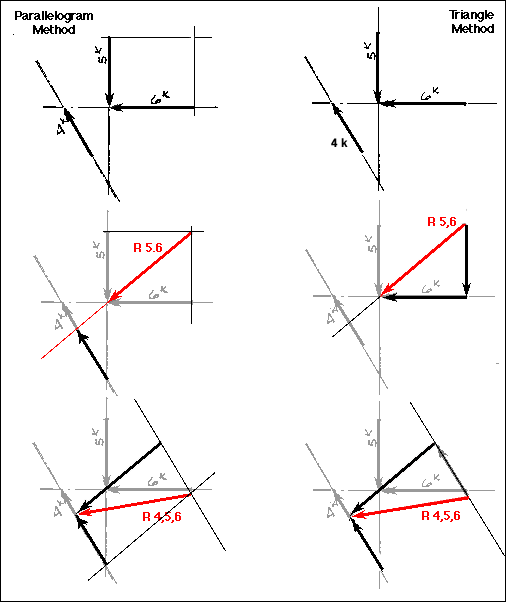## Lecture 8Example Problem

Comparison of Parallel and Triangular methods of force resolution
Given the three forces as indicated, determine the resultant.Solution:
The first thing to do with any graphical problem is to examine the given forces for points of concurrence. In this case the point of obvious concurrence is between the six and five kip forces. These are initially combined. The line of action of this resultant is projected until it meets the line of action of the four kip load. This vector is translated so that the heads are together. Then, depending on the method being used, the resultant of these two forces is determined. Note that the resultant vector MUST be drawn so that all of the heads of the three vectors are together.